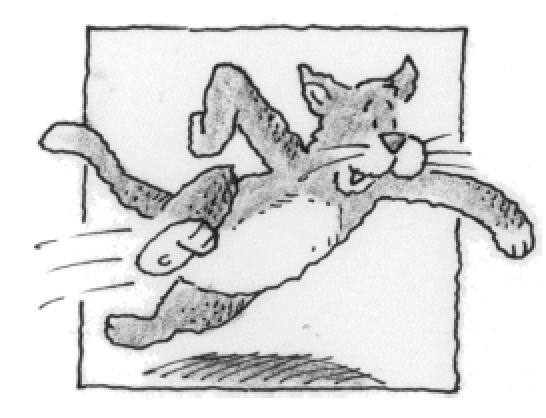### Home > CALC3RD > Chapter Ch5 > Lesson 5.5.1 > Problem5-160

5-160.

Bob’s cat Alice does wind sprints up and down the hallway of his apartment. Suppose that $t$ minutes into her wind sprints, Alice’s velocity (in ft/min) is given by the formula $v(t)=12\operatorname{sin}( \frac { \pi t } { 4 } )$.

1. What is Alice’s acceleration at $t = 6$ minutes?

Acceleration is the derivative of velocity.

2. What is Alice’s displacement between $t = 4$ and $t = 24$?

Displacement is the area under a velocity graph. Total distance is the area under a speed graph.

3. What is the total distance traveled by Alice between $t = 2$ and $t = 8$?

Refer to the hint in part (b).